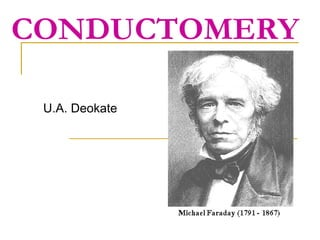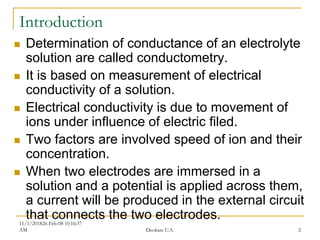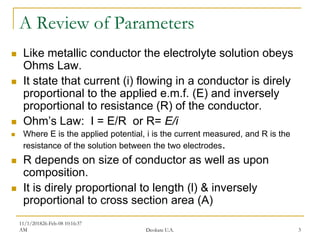Successfully reported this slideshow.

# Conductomery new

12

Share×
1 of 20
1 of 20

# Conductomery new

12

Share

Conductometric titration, Specfic conductance and Molar Conductance, Direct conductometry, Applicaiton

Conductometric titration, Specfic conductance and Molar Conductance, Direct conductometry, Applicaiton

## More Related Content

### Related Books

Free with a 14 day trial from Scribd

See all

### Related Audiobooks

Free with a 14 day trial from Scribd

See all

### Conductomery new

1. 1. CONDUCTOMERY U.A. Deokate
2. 2. 11/1/201826-Feb-08 10:16:37 AM Deokate U.A. 2 Introduction  Determination of conductance of an electrolyte solution are called conductometry.  It is based on measurement of electrical conductivity of a solution.  Electrical conductivity is due to movement of ions under influence of electric filed.  Two factors are involved speed of ion and their concentration.  When two electrodes are immersed in a solution and a potential is applied across them, a current will be produced in the external circuit that connects the two electrodes.
3. 3. 11/1/201826-Feb-08 10:16:37 AM Deokate U.A. 3 A Review of Parameters  Like metallic conductor the electrolyte solution obeys Ohms Law.  It state that current (i) flowing in a conductor is direly proportional to the applied e.m.f. (E) and inversely proportional to resistance (R) of the conductor.  Ohm’s Law: I = E/R or R= E/i  Where E is the applied potential, i is the current measured, and R is the resistance of the solution between the two electrodes.  R depends on size of conductor as well as upon composition.  It is direly proportional to length (l) & inversely proportional to cross section area (A)
4. 4. 11/1/201826-Feb-08 10:16:37 AM Deokate U.A. 4 A Review of Parameters  R α l and R α l/A  R α l/A  R = ρ l/A where ρ constant called resistivity or specific resistance  It is defined as the resistance in ohm of a specimen 1 cm in length and 1 cm in cross section  ρ = RA/ l = ohm x cm2/ cm  i.e. ohm cm  For chemical purposes it is more convenient to discuss the inverse property called the conductance (1/R) expressed in Ω-1 or Siemens (S),  where 1S = 1Ω-1 and 1 μ Ω -1 = 1 μS.
5. 5.  The specific resistance is, thus defined as the resistance of one centimeter cube of a conductor.  The reciprocal of specific resistance is termed the specific conductance or it is the conductance of one centimeter cube of a conductor.  When a gram equivalent of solute is preset in a solution placed between two large parallel electrodes at 1cm apart the conductance is referred as Equivalent conductance.  Molar conductivity is defined as the conductivity of one mole per liter of solution.  Conductivity at infinite dilution: at infinite dilution the ions are independent of each other and they individually contribute to total conductance. 11/1/201826-Feb-08 10:16:37 AM Deokate U.A. 5
6. 6. 11/1/201826-Feb-08 10:16:37 AM Deokate U.A. 6
7. 7. 11/1/201826-Feb-08 10:16:37 AM Deokate U.A. 7 Conductivity measurement  Conductivity is measured with a cell consisting of two platinum electrodes at fixed distance apart.  These electrodes are coated with finely divided electrodeposited platinum black.  The ratio l/A is a property of the cell and is called the cell constant. It is the resistance that is measured with an alternating current to minimize electrolysis.  The catalytic properties of the platinum black allow what ever electrolysis there is to occur so rapidly in both directions that there is no buidup (depletion of anions and cations) of products.  The cell constant is not measured geometrically but by measuring the resistance of a sample solution of known conductivity, usually potassium chloride
8. 8.  Conductometric measurements are usually carried out in a conductance cell, which consists of two parallel sheets of platinum (pt.) as inert electrodes.  The pt. electrodes must be platinized, i.e. Covered by pt.black this will give large surface area which will absorb tiny quantities of electrode reaction products produced during passage of current.  The conductance (G) is directly proportional to the surface area (A) and inversely proportional to the distance between the two electrodes (L ).
9. 9. Instrumentation: 11/1/201826-Feb-08 10:16:37 AM Deokate U.A. 9
10. 10. 1. Conductance cell
11. 11. 11/1/201826-Feb-08 10:16:37 AM Deokate U.A. 11 Conductivity Cell  A conductivity measuring cell is formed by two 1-cm square surfaces spaced 1-cm apart.  Cells of different physical configuration are characterized by their cell constant, K. This cell constant (K) is a function of the electrode areas, the distance between the electrodes and the electrical field pattern between the electrodes.  Since K (cell constant) is a "factor“ which reflects a particular cell's physical configuration, it must be multiplied by the observed conductance to obtain the actual conductivity reading.
12. 12. 11/1/201826-Feb-08 10:16:37 AM Deokate U.A. 12 Application of condutometry  Direct conductivity  In water treatment plant conductivity of water is measured, which is due to ionic compounds dissolved in water.  Increase impurities shows increase conductivity.  Conductivity of water@25OC  Absolute pure water 0.055 µs/cm or 18.3 megohm/cm  Distilled water 0. 5 µs/cm or 2 megohm/cm  D.M. water 5 µs/cm or 200 Kiloohm/cm  TDS in ppm as NaCl = cond. In µs/cm x 0.5  TDS in ppm = cond. In µs/cm x 0.75
13. 13. 11/1/201826-Feb-08 10:16:37 AM Deokate U.A. 13 Drawback of direct conductivity  Non specific  Can not distinguish between different types of ions  Conductivity in aq solution is due to motion of ions and increases with increase in temp.  A small difference in temp make considerable change in conductance of solution.
14. 14. 11/1/201826-Feb-08 10:16:37 AM Deokate U.A. 14 Condutometric titrations  It is the one of the most important application  Various titration such as acid-base titrations, replacement titrations, precipitations and complex formation reaction involving titration are possible  The principle involved is; when a soln of one electrolyte is added to the soln of other the overall conductance will depend on reaction occurring in them.
15. 15. 11/1/201826-Feb-08 10:16:37 AM Deokate U.A. 15 Condutometric titrations  Although water is itself a very poor conductor of electricity, the presence of ionic species in solution increases the conductance considerably.  The conductance depends on the type and concentration of all ions present in solution, as well as the temperature and construction of the conductance-monitoring device.  Thus, monitoring the conductance can provide a sensitive measure of the changes taking place in the ionic composition of a solution, in the course of chemical reaction occurring during conductometric titration.
16. 16. 11/1/201826-Feb-08 10:16:37 AM Deokate U.A. 16 Condutometric titration of strong acid and strong base Change in conductivity during the course of a titration in which HCl is the analyte and KOH is the titrant.  Initially, the measured conductivity will have a relatively high value, As KOH titrant is added, the conductivity decreases, since HCl is converted to KCl and water.  At the equivalence point in the titration, all of the HCl has been neutralized to form KCl, and the conductivity will be at its lowest value.  As KOH is added after reaching the equivalence point, the conductivity will begin to rise again, since KCl and unreacted KOH are both ionized in solution.  The shape of the titration curve obtained is shown
17. 17. 11/1/201826-Feb-08 10:16:37 AM Deokate U.A. 17 Condutometric titration of strong acid and weak base (HCL with ammonia solution)  Initially, the measured conductivity will have a relatively high value,  As ammonia soln as titrant is added, the conductivity decreases, since HCl is converted to NH4Cl and water.  At the equivalence point in the titration, all of the HCl has been neutralized to form NH4Cl, and the conductivity will be at its lowest value.  After the end point the graph become almost horizontal. Volume of titrant added C o n d u c t a n c e
18. 18. 11/1/201826-Feb-08 10:16:37 AM Deokate U.A. 18 Condutometric titration of weak acid and strong base (acetic acid with NaOH)  Initially, the conductance is due to ionization of acetic acid ,  The salt form increases conductance .  At the equivalence point rise in conductance is observed due to hydroxyl ions Volume of titrant added C o n d u c t a n c e
19. 19. 11/1/201826-Feb-08 10:16:37 AM Deokate U.A. 19 Condutometric titration of weak acid and weak base (acetic acid and ammonia solution)  Initially, the conductance is due to ionization of acetic acid ,  The salt form increases conductance .  After the end point the graph become almost horizontal. Volume of titrant added C o n d u c t a n c e
20. 20. 11/1/201826-Feb-08 10:16:37 AM Deokate U.A. 20 Conductometric Titration of mixture of acids (HCL and acetic acid with strong base) Amount of NaOH Solution Added Initially conductance is due to neutralization of strong acid and then rises as the weak acid is converted in to its salts. After the complete neutralization of both acid conductance finally rises more steeply as the excess of alkali (OH) ions are introduced